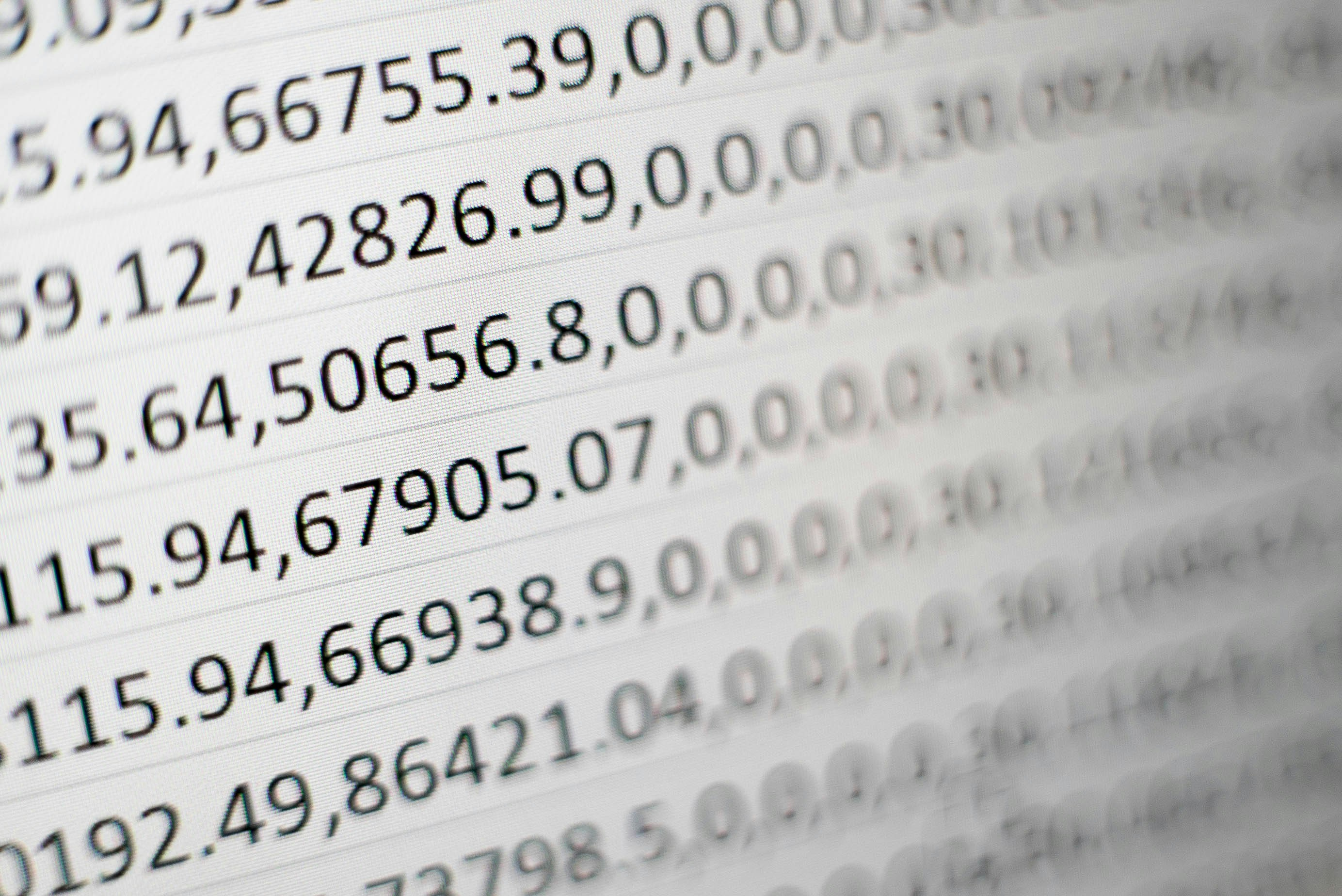In this tutorial, we'll see how to round float values in Pandas.

We'll illustrate several examples related to rounding numbers in DataFrame like:

• round number to 2 decimal places
• round up
• round down
• round float to int
• round number to nearest
• round single column
• round whole DataFrame

If you need to format or suppress scientific notation in Pandas please check: How to Suppress and Format Scientific Notation in Pandas

## Setup

For this article we are going to create Pandas DataFrame with random float numbers. To generate random float numbers in Python and Pandas we can use the following code:

Generating N random float numbers in Python:

``````import random
nums = []
for i in range(10):
x = random.uniform(0.1, 10.0)

nums.append(round(x, 5))
``````

Next we will create DataFrame from the above data:

``````import pandas as pd

data = {'val1': nums[:5], 'val2': nums[5:]}
df = pd.DataFrame(data, columns = ['val1', 'val2'])
``````

DataFrame looks like:

val1 val2
0 3.73156 3.62060
1 0.34162 0.93644
2 1.25441 9.39390
3 3.65596 2.66972
4 3.38249 6.26096

## Step 1: Round up values to 2 decimal places

Let's start by rounding specific column up to 2 decimal places.

``````df['val1'].round(decimals = 2)
``````

or simply:

``````df['val1'].round(2)
``````

The result is a Series from the rounded data:

``````0    3.73
1    0.34
2    1.25
3    3.66
4    3.38
Name: val1, dtype: float64
``````

So we can see that data was rounded up. For example:

``````3.65596
``````

become

``````3.66
``````

## Step 2: Round down numbers in specific column

Next let's cover how to round down a column in Python and Pandas.

We will use `np.floor` for rounding down:

``````import numpy as np
df['val1'].apply(np.floor)
``````

result is:

``````0    3.0
1    0.0
2    1.0
3    3.0
4    3.0
Name: val1, dtype: float64
``````

If you need decimal precision or custom rounding down please refer to step 4.

## Step 3: Round up values

We can use `np.ceil` to round up numbers in Pandas:

``````import numpy as np
df['val1'].apply(np.ceil)
``````

result is:

``````0    4.0
1    1.0
2    2.0
3    4.0
4    4.0
Name: val1, dtype: float64
``````

If you need decimal precision or custom rounding up please refer to the next step.## Step 4: Round down values with decimal precision

We can define custom function in order to round down values in Pandas with decimal precision.

This allow us to use any custom logic to round numbers in Pandas:

``````def my_floor(a, precision=0):
return np.true_divide(np.floor(a * 10**precision), 10**precision)

df['val1'].apply(my_floor, precision=3)
``````

The result is round down float to the 3rd decimal place:

``````0    3.731
1    0.341
2    1.254
3    3.655
4    3.382
Name: val1, dtype: float64
``````

## Step 5: Round number to nearest integer

We can round floats to nearest integer in Pandas by combining:

• `round()`
• `astype()`

The code is:

``````df['val1'].round(0).astype(int)
``````

and the result is:

``````0    4
1    0
2    1
3    4
4    3
Name: val1, dtype: int64
``````

## Step 6: Round values in the whole DataFrame

So far we've worked with single columns. If you need to apply rounding operations over the whole DataFrame we can use method `round()`:

``````df.round(3)
``````

The result is rounding up to 3 decimal places:

val1 val2
0 3.732 3.621
1 0.342 0.936
2 1.254 9.394
3 3.656 2.670
4 3.382 6.261

## Conclusion

To summarize, in this article, we've seen examples of rounding numbers and values in Pandas. We briefly described rounding up and down.

And finally, we've seen how to apply rounding on specific columns or the whole DataFrame.